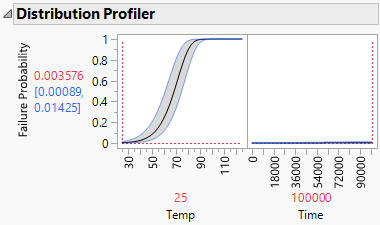Design of Experiments Guide > Accelerated Life Test Designs > Example of Augmenting an Accelerated Life Test Design
Publication date: 05/24/2021

# Example of Augmenting an Accelerated Life Test Design

This example shows how to use the Accelerated Life Test Design platform to augment an existing design. In this example, 150 capacitor units were tested across three temperatures (85o, 105o, and 125o Celsius) for 1500 hours. The results are recorded in the Capacitor ALT.jmp sample data table. The resulting model is used to predict the fraction of the population that is failing at 100,000 hours at a normal usage temperature of 25o Celsius.

#### Review Current Predictions

1. Select Help > Sample Data Library and open Design Experiment/Capacitor ALT.jmp.

2. Click the green triangle to run the Fit Life by X table script.

3. In the Distribution Profiler, found in the Comparisons outline on the Distribution tab, change the factor settings:

Click 105 above Temp and change it to 25.

Click 750.5 above Hours and change it to 100,000.

Figure 22.10 Distribution Profiler for Capacitor Model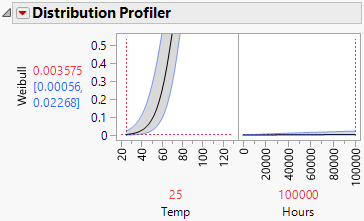Based on your current study, the predicted fraction of the capacitor population that fails at 25o at 100,000 hours is 0.003575, with a 95% confidence interval of 0.00056 to 0.02268. You want to estimate the fraction of failures more precisely. To decrease the width of the confidence interval, a measure of the precision of your estimate, you can augment your study with additional tests.

#### Augment the Design

You want to augment your design to obtain a more precise estimate of the predicted fraction of failures. Your original design used temperature settings of 85, 105, and 125. In your augmented design, you want to test at temperature values of 90, 110, and 125. Note that two of these settings are new. Augment the design with optimally selected runs:

1. Select DOE > Special Purpose > Accelerated Life Test Design.

2. Select One accelerating factor, select Continuous Monitoring, and click Continue.

3. Enter Temp for Factor Name.

4. Enter 5 for Number of Levels.

Even though your augmented runs span only three levels (90, 110, and 125), you must also specify the levels used in the original experiment for a total of five levels. The Factor Transformation is set to Arrhenius Celsius by default.

5. Enter 25 for both Low Usage Condition and High Usage Condition.

6. Enter 85 for the Low Test Condition and 125 for the High Test Condition.

7. Click Continue.

8. Enter 85, 90, 105, 110, and 125 for the Temp Level Values.

There are three levels from the original experiment (85, 105, and 125). The augmented design has two new levels (90 and 110) and one of the levels from the first experiment (125).

Note: All levels must be listed.

9. Ensure that Weibull is selected as the Distribution Choice.

10. Under Prior Mean, select Specify Intercept. Enter your current acceleration model parameter estimates from the Fit Life by X Estimates outline, found in the Weibull Results outline on the Statistics tab.

Figure 22.11 Parameter Estimates and Fitted Model from Weibull Results Outline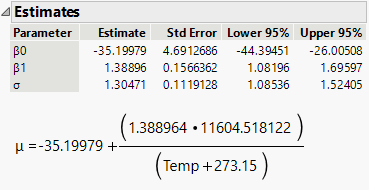Enter -35.200 for Intercept.

Enter 1.389 for Activation Energy (Temp).

This is an estimate of the activation energy and is the coefficient of the inverse temperature, measured in degrees Kelvin, multiplied by Boltzmann’s constant.

Enter 1.305 for 1/β .

For the Weibull distribution, JMP uses a parameterization that depends on a location parameter μ and scale parameter σ. In terms of the usual α and β parameterization, the scale parameter is σ = 1/β. See Weibull.

In the Accelerated Life Test Plan window, you can specify uncertainty about your prior means by selecting the Specify prior uncertainty option. In this example, you do not specify prior uncertainty. Your design is created assuming that the values specified in the Prior Means outline are the true parameter values.

11. Under Design Choices, enter 1500 for Length of test.

The test is conducted over 1500 hours, which was the length of the original design.

12. Enter 250 for Number of units under test.

The previous experiment tested 150 units, and the augmented experiment tests an additional 100 units, for a total of 250 units.

Figure 22.12 Completed Design Details Window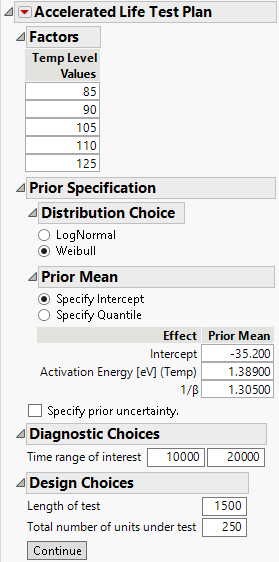13. Click Continue.

14. Under Candidate Runs enter 50 as the minimum number of units for the temperatures of 85, 105, and125. These represent the runs already completed.

15. Click the Accelerated Life Test Plan red triangle and select ALT Optimality Criterion > Make Failure Probability Optimal.

16. Click Make Design.

The optimal experimental design appears along with other results.

Figure 22.13 Optimal Design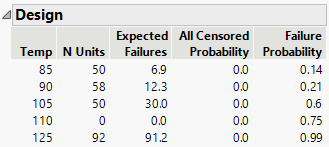The optimal design is computed based on the levels of the test runs, the minimum number of units under test, the total number of units to be tested (this is the information in the Candidate Runs outline), and other information that you specified. The optimal design consists of testing the specified number of units at each temperature level:

50 units at 85oC. Since the previous experiment already tested 50 units at 85oC, no additional units are needed.

58 units at 90oC.

50 units at 105oC. Since the previous experiment tested 50 units at 105oC, no additional units are needed.

0 units at 110oC. This level is not needed.

92 units at 125oC. Since the previous experiment tested 50 units at 125oC, 42 additional units are needed.

#### Compare the Augmented Design to the Original Study

1. In the Distribution Profiler, enter the normal use condition of 25 for Temperature and 100,000 for Time. The estimate of the fraction of the population that is failing is 0.00357, with a 95% confidence interval of 0.00089 to 0.014525. This interval is narrower than the interval from the initial experiment, which was from 0.00056 to 0.02268 (Figure 22.10).

Figure 22.14 Distribution Profiler for Temp = 25 and Time = 100000